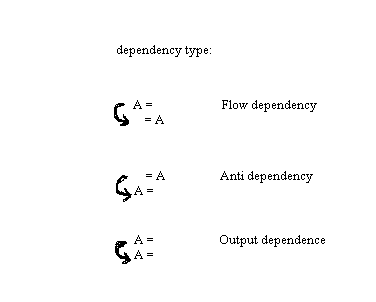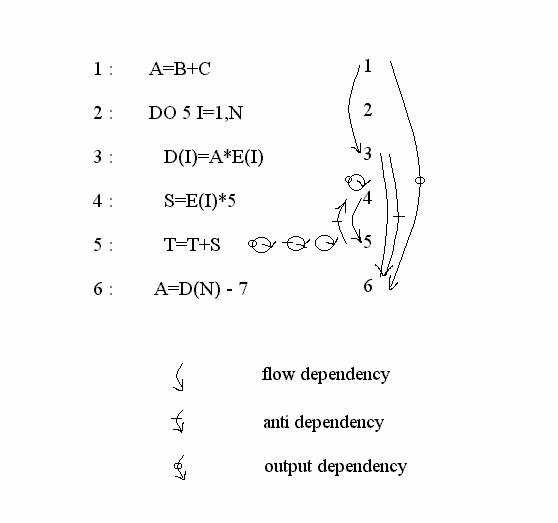## Dependency graphs

A program is a collection of statements, the ordering and scheduling of which depends on dependence constraints. Dependencies are broadly classified into two categories:

1. Data Dependencies: when statements compute data that are used by other statements.

2. Control Dependencies: are those which arise from the ordered flow of control in a program.

A dependence graph can be constructed by drawing edges connect dependent operations. These arcs impose a partial ordering among operations that prohibit a fully concurrent execution of a program. The use-definition chaining is a form of dependency analysis but it leads to overly conservative estimates of data dependence. Four kind of dependence may exist between statement number i and j on a common control path:Figure 1 : Dependency types

1. Flow Dependence: Sj is flow-dependent on Si if a value of a variable used by Sj was computed by Si.

2. Antidependence: Sj is antidependent on Si if a value of a variable used by Si is recomputed by Sj.

3. Output-Dependence: Sj is output-dependent on Si if both compute the same variable and value of the variable from Sj has to be stored after that from Si

4. Control-Dependence: Sj is control-dependent on a conditional statement Si if its execution of Si and the path chosen after that( Si must execute before Sj).

Example of Dependency Analysis

Figure 2 shows a FORTRAN program code and dependency analysis based on that program segment. Variable A in statements 1 and 3 is an example of flow dependence in which a variable is defined in one statement and used in a subsequent statement. Anti Dependence is mirror image of flow dependence, it occurs when a variable is used in an earlier statement and then reassigned in a subsequent statement. An example is variable A in statement 3 and 6 of figure 2. Output dependence occurs when a variable is assigned more than once in different statements as in statement 1 and 6 of figure 2.Figure 2: A Program in FORTRAN with corresponding dependency graph

Use of Dependency Analysis in Compiler Design

The basic idea behind dependency graphs is for compiler to look for various kinds if dependence among statements to prevent their execution in wrong order i.e. the order that changes the meaning of the program. This helps it to identify various parallelisable components in the program.

Wireless Networking: Introduction to Bluetooth and WiFi